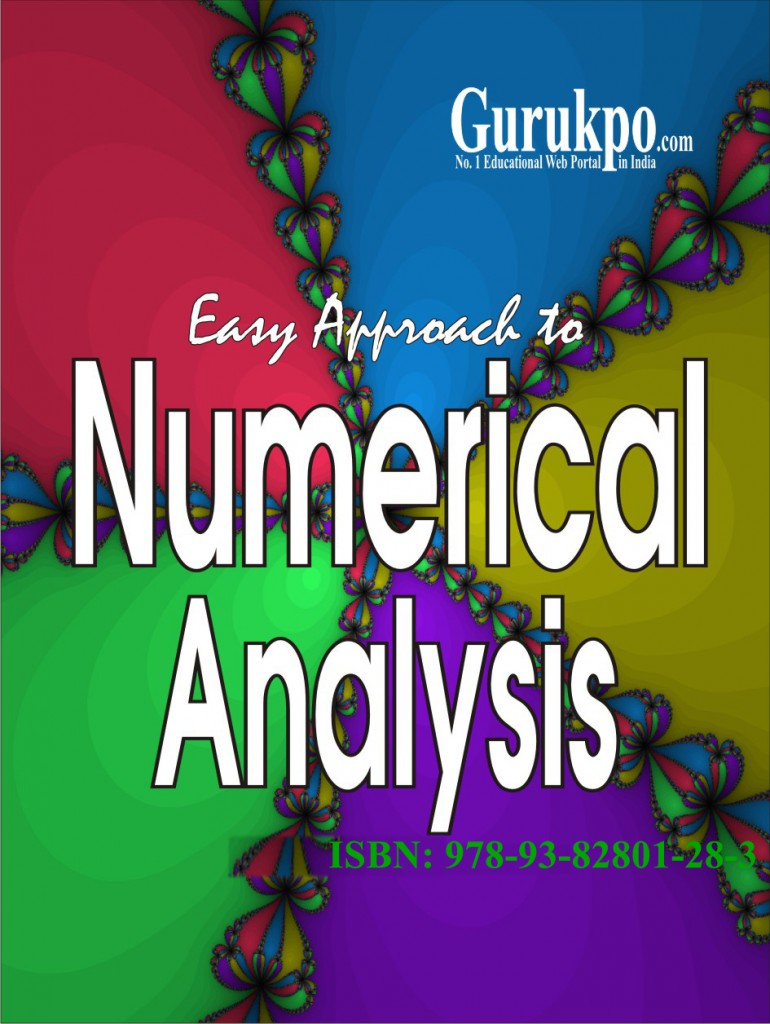## Mathematical Methods for Numerical Analysis and Optimization

Computer arithmetics and errors. Algorithms and programming for numerical solutions. The impact of parallel computer : introduction to parallel architectures. Basic algorithms Iterative solutions of nonlinear equations : bisection method, Newton-Raphson method, the Secant method, the method of successive approximation. Solutions of simultaneous algebraic equations, the Gauss elimination method. Gauss-Seidel Method, Polynomial interpolation and other …